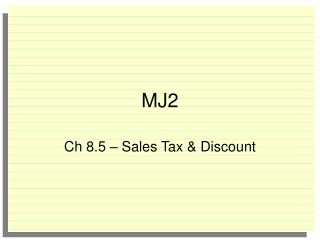DownloadDownload PresentationMJ2

# MJ2

Télécharger la présentation## MJ2

- - - - - - - - - - - - - - - - - - - - - - - - - - - E N D - - - - - - - - - - - - - - - - - - - - - - - - - - -
##### Presentation Transcript

1. MJ2 Ch 8.5 – Sales Tax & Discount

2. / / / • • • • • 0 0 0 0 0 1 1 1 1 1 2 2 2 2 2 3 3 3 3 3 4 4 4 4 4 5 5 5 5 5 6 6 6 6 6 7 7 7 7 7 8 8 8 8 8 9 9 9 9 9 1. A recipe calls for 2 cups of flour to make 24 cookies. How many cups of flour would it take to make 39 cookies? 7 / 4 24 • n = 2 • 39 24 n = 78 Bellwork – You do not have to write the question – Grid your answer

3. Assignment Review • Practice Skills Workbook Lesson 8.4

4. Before we begin… • Please take out your notebook and get ready to work… • In the last lesson we looked percent of change…that is the percent of increase or decrease • In today’s lesson we will look at markup, discount and sales tax…discount represents a decrease and markup & sales tax represent increases…

5. Objective • Students will solve problems involving discount and sales tax.

6. Markup & Discount • When a store sells an item for more than it paid, the extra money is used to cover expenses and to make a profit. • The increasein price is called markup. The percent of markup is a percent of increase. • The amount the customer pays is called the selling price • When a store has a sale, the amount by which the regular price is reduced is called discount. The percent of discount is a percent of decrease

7. Calculating Markup & Discount • Markup– to calculate markup, multiply the original price by the percent of increase, and thenaddthat amount to the original price to get the selling price. • Discount – to calculate discount, multiply the original price by the percent of decrease and then subtract that amount from the original price to get the sale price.

8. Example - Markup • A store is selling a jacket for 25% over cost. The cost is \$35. What is the selling price? \$35 x 25% = \$8.75 \$35 + \$8.75 = \$43.75 Selling Price

9. Your Turn • In the notes section of your notebook calculate the selling price • Computer: \$700 Markup: 30% • CD Player: \$120 Markup: 20% • Jeans: \$25 Markup: 45%

10. Example - Discount • A computer sells for \$1,200. The store puts the computer on sale for 30% off. What is the sale price? \$1200 x 30% = \$360 \$1200 - \$360 = \$840 Sale price

11. Your Turn • In the notes section of your notebook calculate the sale price • Video game: \$75 Discount: 25% • Skateboard: \$119.95 Discount: 30% • Television: \$675.50 Discount 35%

12. Sales Tax • Sales tax is an additional amount of money charged on items that people buy. The local, state & federal government receives this money. • The total cost of an item is the price plus the sales tax. • NOTE: You do not pay tax on the price of an item before discount. You pay the tax on the discounted amount!

13. Example – Sales Tax • What is the total cost of a sweatshirt if the original price is \$42 and the sales tax is 5½%? • In this instance you calculate the sales tax by multiplying the \$42 by 5.5% and then add that amount to the \$42 42 ● 5.5% = 2.31 (Tax amount) 42 + 2.31 = \$44.31 Total price

14. Your Turn • In the notes section of your notebook calculate the total price: • \$2.95 notebook; 5% tax • \$46 shoes; 2.9% tax • \$178.90 video game; 3.75% tax

15. Summary • In the notes section of your notebook summarize the key concepts covered in today’s lesson • Today we discussed • Markup, discount, & sales tax

16. Assignment • Text p. 356 # 11 - 20 Reminder: • This assignment is due tomorrow • I do not accept late assignments • I do not accept answers only for any assignment (no work = no credit)